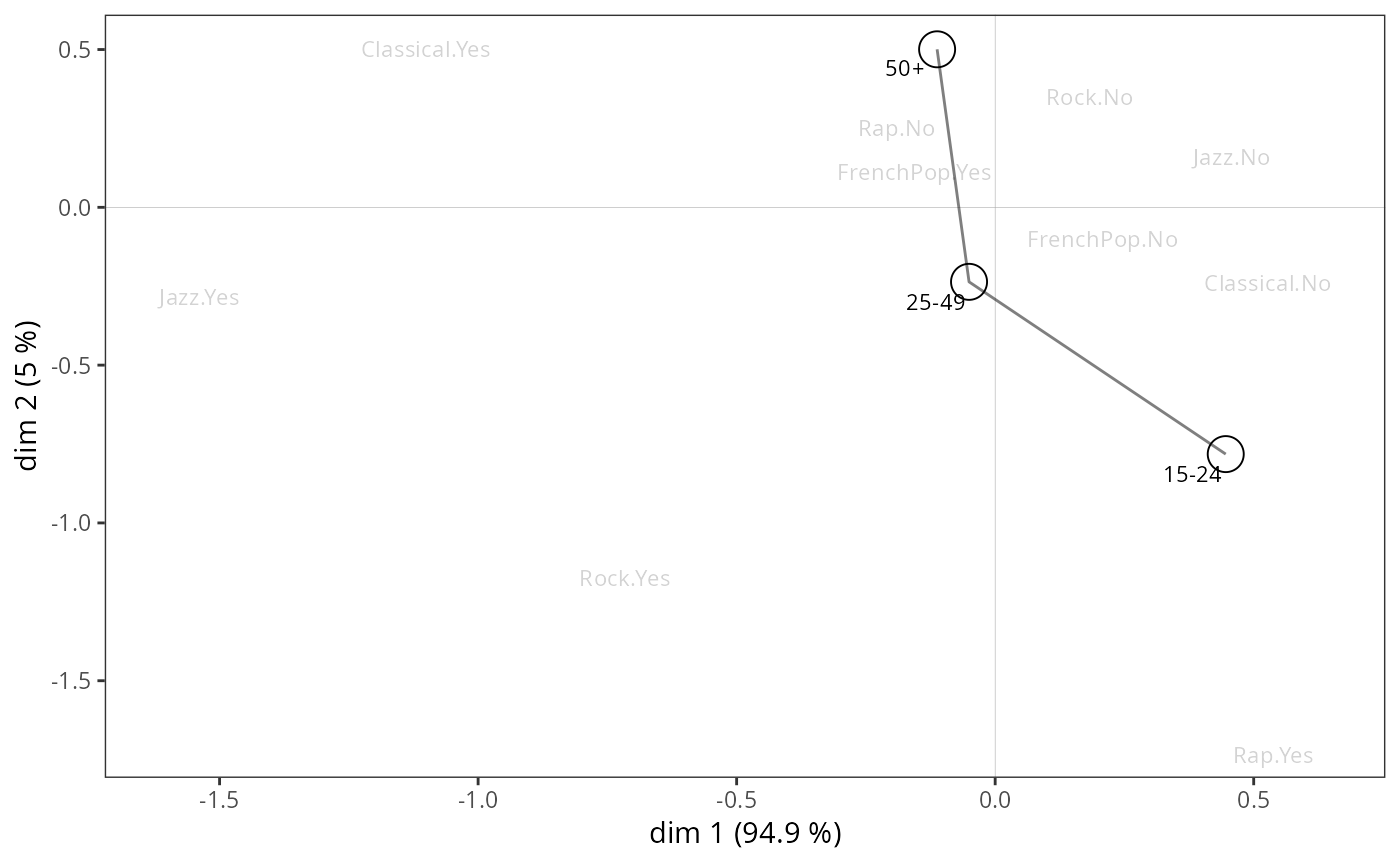Adds a categorical supplementary variable to a MCA cloud of variables.

ggadd_supvar(p, resmca, var, sel = 1:nlevels(var), axes = c(1,2),
col = "black", shape = 1, prop = NULL, textsize = 3, shapesize = 6,
segment = FALSE, vname = NULL)

## Arguments

p

ggplot2 object with the cloud of variables

resmca

object of class MCA, speMCA, csMCA, stMCA or multiMCA.

var

Factor. The categorical supplementary variable. It does not need to have been used at the MCA step.

sel

Numeric vector of indexes of the categories of the supplementary variable to be added to the plot. By default, labels are plotted for every categories.

axes

numeric vector of length 2, specifying the components (axes) to plot. Default is c(1,2).

col

Character. Color of the shapes and labels of the categories. Default is black.

shape

Symbol to be used in addition the the labels of categories (default is 1). If NULL, only labels are plotted.

prop

If NULL, the size of the labels (if shape=NULL) or the shapes (otherwise) is constant. If 'n', the size is proportional the the weights of categories; if 'vtest1', the size is proportional to the test values of the categories on the first dimension of the plot; if 'vtest2', the size is proportional to the test values of the categories on the second dimension of the plot; if 'cos1', the size is proportional to the cosines of the categories on the first dimension of the plot; if 'cos2', the size is proportional to the cosines of the categories on the second dimension of the plot; if 'cos12', the size is proportional to the total cosines of the categories on the two dimensions of the plot.

textsize

Size of the labels of categories if shape is not NULL, or if shape=NULL and prop=NULL. Default is 3.

shapesize

Size of the shapes if prop=NULL, maximum size of the shapes in other cases. Default is 6.

segment

Logical. Should one add lines between categories ? Default is FALSE.

vname

A character string to be used as a prefix for the labels of the categories. If NULL (default), no prefix is added.

## Value

a ggplot2 object

## Author

Nicolas Robette

ggcloud_variables, ggadd_supvars, ggadd_ellipses, ggadd_kellipses, ggadd_density, ggadd_interaction, ggsmoothed_supvar, ggadd_chulls, ggadd_corr

## Examples

# specific MCA of Music example data set
data(Music)
junk <- c("FrenchPop.NA", "Rap.NA", "Rock.NA", "Jazz.NA", "Classical.NA")
mca <- speMCA(Music[,1:5], excl = junk)
# adds Age as a supplementary variable
# onto the cloud of variables
p <- ggcloud_variables(mca, col = "lightgrey", shapes = FALSE)
ggadd_supvar(p, mca, Music\$Age, segment = TRUE)